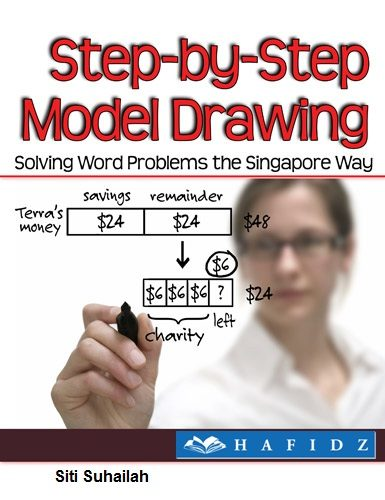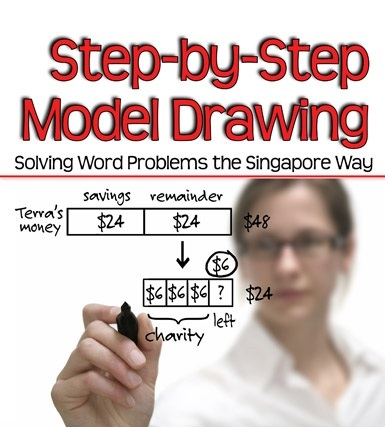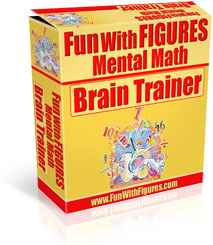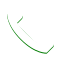Ever teach your child Maths Problem Sums using the Model Drawing but discover that you do  not know how to draw the diagram? If you have, you might want to know that you are not alone. Many parents are facing the same problem as you simply because you were not this way when you were young.

Model Drawing has since been widely used in the teaching of students in primary schools in Singapore. They are introduced to the method from as young as Primary One.

Students typically find word problems difficult due to several reasons:• they are weak in their Mathematical language;
• they have limited understanding of the arithmetic operations;
• they are unable to relate the known(s) to the unknown(s) when the problem structure is difficult to understand;
• they are unable to analyse problem situations.

This method is especially useful when: you teach kids who respond better to visual stimuli (e.g. pictures, drawings, etc); you try to provide math homework help but the conventional methods do not really work well with your kids; and your kids has not learnt algebra yet and solving the math problems with algebra is not an option.

However, without proper guidance, you may not be able to experience the full benefits of the Model Drawing. The Model Drawing essentially becomes a good entry level tool to help the your child to understand and break the questions down into component parts making solving and learning math easy.What is a Model Drawing ?The model approach requires students to draw rectangular boxes to represent part-whole relationships and Math Value. By drawing such blocks, they can visualize the math problems more clearly and are able to make tacit knowledge explicit. Word problem solving is a major part of the curriculum in Primary Mathematics in Singapore, both known and unknown values, in the Maths Problem Sums.This technique of model building is a visual way of picturing a situation. Instead of forming simultaneous equations and solving for the variables, model building involves using blocks or boxes to solve the problem. The power of using models can be best illustrated by problems, often involving fractions, ratios or percentages, which appear difficult but if models can be drawn to show the situation, the solution becomes clearer, sometimes even obvious.

Previous

••Need Help?